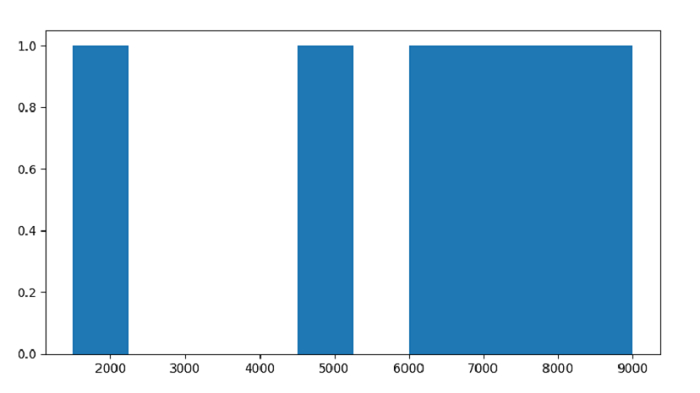# Python - Plot a Histogram for Pandas Dataframe with Matplotlib?

Histogram is a representation of the distribution of data. To plot a Histogram, use the hist() method. At first, import both the libraries −

import pandas as pd
import matplotlib.pyplot as plt

Create a DataFrame with 2 columns −

dataFrame = pd.DataFrame({
"Car": ['BMW', 'Lexus', 'Tesla', 'Mustang', 'Mercedes', 'Jaguar'],"Reg_Price": [7000, 1500, 5000, 8000, 9000, 6000]
})

Plot a Histogram for Registration Price column −

plt.hist(dataFrame["Reg_Price"])

## Example

Following is the code −

import pandas as pd
import matplotlib.pyplot as plt

# creating dataframe
dataFrame = pd.DataFrame({
"Car": ['BMW', 'Lexus', 'Tesla', 'Mustang', 'Mercedes', 'Jaguar'],"Reg_Price": [7000, 1500, 5000, 8000, 9000, 6000]
})

# plot a histogram for Registration Price column
plt.hist(dataFrame["Reg_Price"])
plt.show()

## Output

This will produce the following output −